# Test: Work, Energy & Power - 2

## 30 Questions MCQ Test Physics For JEE | Test: Work, Energy & Power - 2

Description
Attempt Test: Work, Energy & Power - 2 | 30 questions in 60 minutes | Mock test for NEET preparation | Free important questions MCQ to study Physics For JEE for NEET Exam | Download free PDF with solutions
QUESTION: 1

### A wheel is at rest. Its angular velocity increases uniformly and becomes 80 radian per second after 5 second. The total angular displacement is :

Solution:

If the angular velocity increases uniformly, then the angular acceleration must be constant.

Hence the formula,

s= ut + 0.5 a t2

Where.

u= initial angular velocity

a= angular acceleration (i.e = 80/5=16 rad/s^2)

S= angular displacement

t= time taken

Now.

s= 0 + 0.5× 16 * 52 = 200

Hence angar displacement = 200 radians

QUESTION: 2

### A wheel is subjected to uniform angular a cceleration about its axis. Initially its angular velocity is zero. In the first 2 sec, it rotates through an angle q1 ; in the next 2 sec, it rotates through an additional angle q2. The ratio of q2 / q1 is-

Solution:

From the second equation of motion we get that
Q1 = 0 + ½ a x 4
Where a is constant angular acceleration
Thus Q1 = 2a
Similarly Q2 = 0 + ½ x a x 16
Thus Q2 = 8a
We get Q2 / Q1 = 4

QUESTION: 3

### The linear and angular acceleration of a particle are 10 m/sec2 and 5 rad/sec2respectively it will be at a distance from the axis of rotation -

Solution:

a = rα (where α is angular acceleration = 5 & a is linear acceleration = 10)

QUESTION: 4

A wheel having a diameter of 3 m starts from rest and accelerates uniformly to an angular velocity of 210 r.p.m. in 5 seconds. Angular acceleration of the wheel is -

Solution: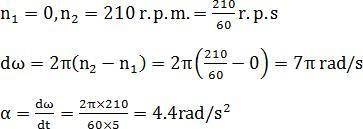QUESTION: 5

The second’s hand of a watch has length 6 cm. Speed of end point and magnitude of difference of velocities at two perpendicular positions will be

Solution: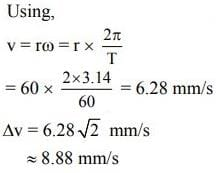QUESTION: 6

The ratio of angular speed of hours hand and seconds hand of a clock is-

Solution:

The angular speed of second hand = 2/ 60rad/sec and angular speed of hour hand = 2/ 12 rad/hr
= 2/ 12 x 60 x 60 rad/sec
Hence the ratio is 1: 720

QUESTION: 7

The angular velocity of earth about its axis of rotation is-

Solution:

Angular velocity = 2π/time period
Time period of earth is one day means 24×60×60

QUESTION: 8

A stone of mass 0.5 kg tied with a string of length 1 metre is moving in a circular path with a speed of 4 m/sec. The tension acting on the string in Newton is-

Solution: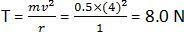QUESTION: 9

Two bodies A & B rotate about an axis, such that angle qA (in radians) covered by first body is proportional to square of time, & qB (in radians) covered by second body varies linearly. At t = 0, qA = qB = 0. If A completes its first revolution in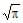sec. & B needs 4p sec. to complete half revolution then; angular velocity wA : wB at t = 5 sec. are in the ratio

Solution:
QUESTION: 10

A car of mass 1000 kg moves on a circular track of radius 20 m. if the coefficient of friction is 0.64, what is the maximum velocity with which the car can be moved?

Solution:

Using the formula Vmax = √urg taking coefficient of friction = (u) in this formula. Hence we have radius and also know gravitational constant. Solving the above question
Vmax = √u*√r*√g
u = 0.64
g = 9.8
r = 20
Vmax = √9.8*√20*√0.64
= √125.44 = 11.2m/s

QUESTION: 11

The earth, radius 6400 km, makes one revolution about its own axis in 24 hours. The centripetal acceleration of a point on its equator is nearly -

Solution:

The time period of earth’s rotation is,
T = 24 h = 86400 s
Angular velocity, ω = 2π/T
Radius of earth, r = 6400 km
=> 6.4 x 106 m
Now, centripetal acceleration = ω2r
=> (2π/T)2r = 0.034 m/s2

QUESTION: 12

A stone of mass 0.1 kg tied to one end of a string 1.0 m long is revolved in a horizontal circle at the rate of 10/p revolution per second. Calculate the tension of the string ?

Solution: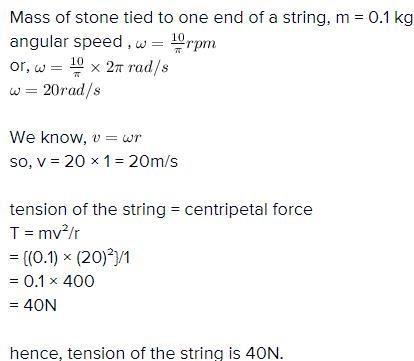QUESTION: 13

A coin placed on a rotating turn table just slips if it is at a distance of 40 cm from the centre if the angular velocity of the turntable is doubled, it will just slip at a distance of

Solution: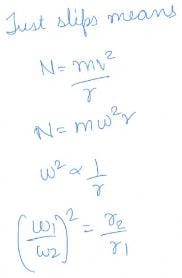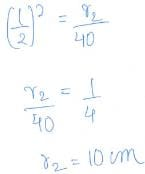QUESTION: 14

A particle moves in a circle of radius 25 cm at two revolutions per second. The acceleration of particle in m/s2 is -

Solution:

The options should be changed to:

1. π2
2. 2
3. 2
4. 2

2 rev/sec means → ω = 2πn = 2π rad/sec
Use the formula of Acceleration a = rω2, where r = 0.25 cm
On substituting and calculating, we get
Acceleration a = 4π2 m/s2

QUESTION: 15

The graphs below show angular velocity as a function of time. In which one is the magnitude of the angular acceleration constantly decreasing?

Solution:

As we know slope at any point on velocity time curve give us acceleration that means we have to choose the curve in which slope on curve is decreasing with time. The graph of angular velocity gives the angular acceleration. In option no 'a' we can see that the graph is constantly decreasing. So, angular acceleration will also constantly decrease.

QUESTION: 16

A particle moves along a circle of radius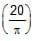m with constant tangential acceleration. If the velocity of the particle is 80 m/s at the end of the second revolution after motion has begun, the tangential acceleration is :

Solution: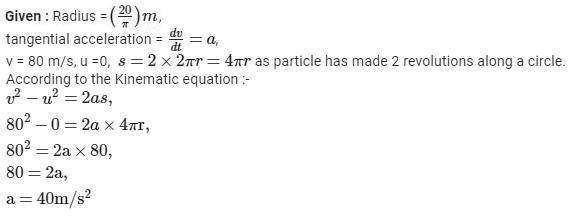QUESTION: 17

A particle moves with deaceleration along the circle of radius R so that at any moment of time its tangential and normal accelerations are equal in moduli. At the initial moment t = 0 the speed of the particle equals v0, then:

(i) the speed of the particle as a function of the distance covered s will be

(A) v = v0 e_s/R (B) v = v0es/R (C) v = v0e_R/s (D) v = v0eR/s

(ii) the total acceleration of the particle as function of velocity and distance covered

(A) a =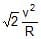(B) a =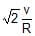(C) a =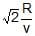(D) a =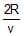Solution:

(i)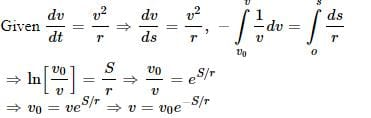(ii)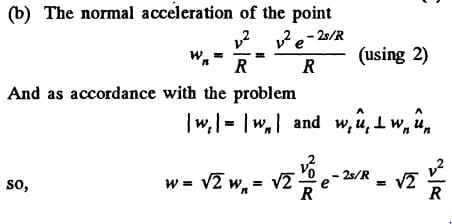QUESTION: 18

If angular velocity of a disc depends an angle rotated q as w = q2 + 2q, then its angular acceleration a at q = 1 rad is

Solution: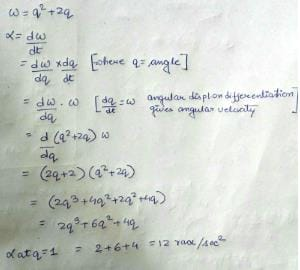QUESTION: 19

A particle is going in a uniform helical and spiral path separately as shown in figure with constant speed.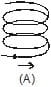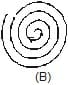Solution:

The centripetal acceleration is inversely proportional to the radius of the circle. In case (A) the spiral path has same radius for every circle, so the particle will have a constant centripetal acceleration but in case (B) the radius of the circles goes on decreasing which results in decrease in centripetal acceleration of the particle.

QUESTION: 20

If the radii of circular paths of two particles of same masses are in the ratio of 1 : 2, then in order to have same centripetal force, their speeds should be in the ratio of :

Solution: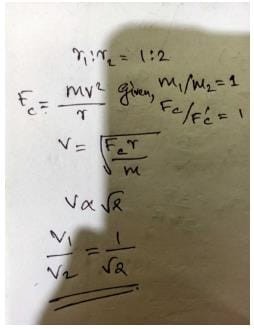QUESTION: 21

A rigid body of mass m is moving in a circle of radius r with a constant speed v. The force on the body is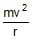and is directed towards the centre. What is the work done by this force in moving the body over half the cirumference of the circle.

Solution: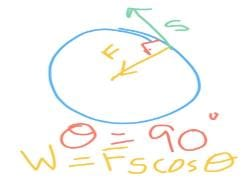Force = mv2/r ( towards the centre )
displacement = 2r
Work done = FScosѲ
here ,
= mv2/r × s × cos 90

QUESTION: 22

A body travels through a distance of 10 m on a straight line, under the influence of 5 N . If the work done by the force is 25J, the angle between the force and displacement is-

Solution:

We Know that,
Work = Force × displacement × cosine of angle between Force and displacement
Given Work (W) = 25J
Displacement (s) = 10 m
Force (F) = 5 N
Let angle between Force and displacement be θ
so W=F × s × cos θ
=> cos θ = 1/2
=>cos θ = cos 60
Hence angle between Force and displacement is 60 degree

QUESTION: 23

A blocks is moved from rest through a distance of 4m along a straight line path. The mass of the blocks is 5 kg. and the force acting on it is 20 N . If the kinetic energy acquired by the block be 40J, at what angle to the path the force is acting-

Solution: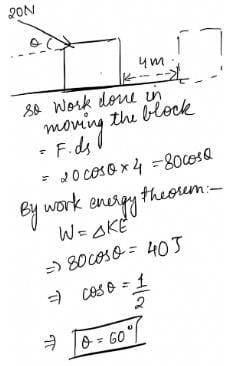QUESTION: 24

The work done in pushing a block of mass 10 kg from bottom to the top of a frictionless inclined plane 5 m long and 3 m high is- (g = 9.8 m/sec2)

Solution:

According to Work Energy Theorem
W = -∆E
So, W = mgh = 294 N

QUESTION: 25

A force F = (3x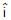+ 4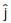) Newton (where x is in metres) acts on a particle which moves from a position (2m, 3m) to (3m, 0m). Then the work done is

Solution: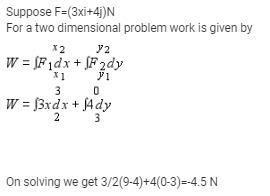QUESTION: 26

If a man increases his speed by 2 m/sec, his K.E. is doubled. The original speed of the man is-

Solution:

Assume initial speed of man = u and kinetic energy = K
Final speed of man = (u+2) then kinetic energy = 2K. Also,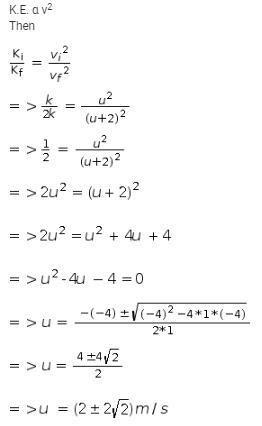QUESTION: 27

A block of mass m is suspended by a light thread from an elevator. The elevator is accelerating upward with uniform acceleration a. The work done by tension on the block during t seconds is :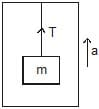Solution:

A block of mass m is suspended by a light thread from an elevator. The elevator is accelerating upward with uniform acceleration ‘a’. We know, Newton's law doesn't valid in non-inertial frame of reference. So, we have to add Pseudo force just opposite direction of it motion to make inertial frame of reference.
So, tension in the thread = mg + ma → [Pseudo force]
Tension = m(g + a)
Now, we have to find displacement of elevator.
Use formula, S = ut + 1/2 At2
Here A is net acceleration acting on the elevator.
Here u = 0, A = a [ upward direction]
So, S = 0 + 1/2 at2
Hence, displacement is 1/2at2
Now, work done = Tension x displacement = m(g + a) x 1/2 at2
= 1/2 m(g + a)at2

QUESTION: 28

In a typical power station, amid a series of energy transfers and conversions, about 70% of the energy input gets wasted in the form of thermal energy, the efficiency of such a power station is about

Solution:

If 70% is wasted then 30% is used, so, the efficiency becomes 30/100 = 0.3

QUESTION: 29

A body of mass m accelerates uniformly from rest to a speed v0 in time t0. The work done on the body till any time t is

Solution:

Acceleration is uniform
vo = 0 + ato
a = vo/to (equation 1)
K.E. of the body at time t = ½ mv2 and v=at
= ½ m(at)02
From equation 1
= ½ mv2t2/to

QUESTION: 30

A light spring of length 20 cm and force constant 2 kg/cm is placed vertically on a table. A small block of mass 1 kg falls on it. The length h from the surface of the table at which the ball will have the maximum velocity is -

Solution: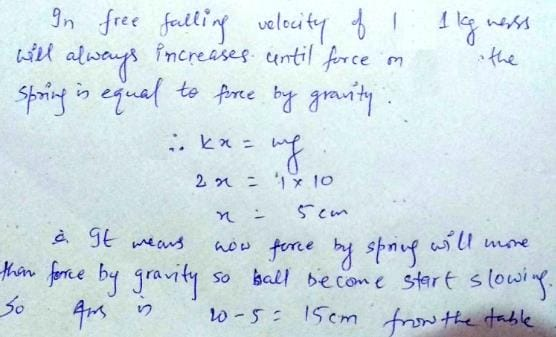Use Code STAYHOME200 and get INR 200 additional OFF Use Coupon Code